2018-05-16

## 使用机器学习中的决策树，logistics，SVM以及随机森林4种方式处理客户丢失的问题（实际为分类问题）

### 一、读取数据

``````import pandas
# 读取csv数据，并指定第0列为索引，第0行为栏位
df = pandas.read_csv('customer_churn.csv', index_col=0, header = 0)
# 打印前几行数据
print(df.head())
# 打印栏位
print(df.columns)``````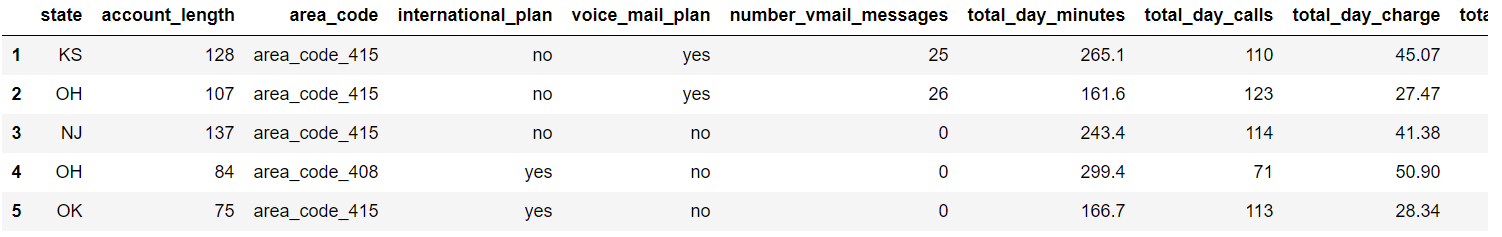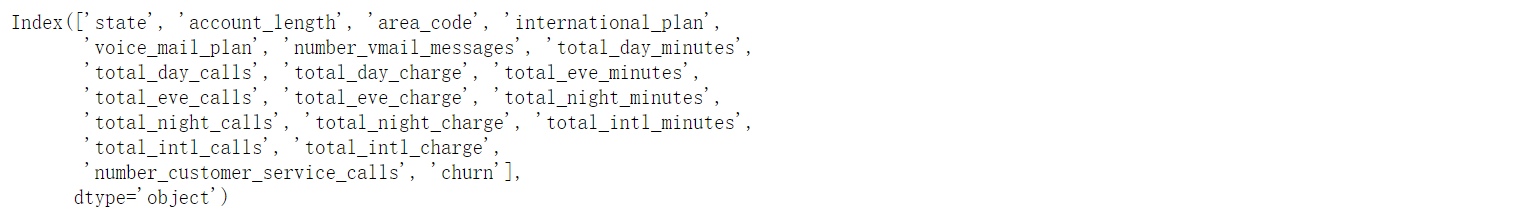1. state 州信息
2. account_length 账户长度
3. area_code 地域代码
4. international_plan 是否有国际计划
5. ...略

### 二、数据清理

``````# 提取从第4列开始到最后一列的所有行的数据并附给新变量
df = df.iloc[:,3:]
# 打印前几行数据
print(df.head())
# 使用循环加匿名函数的方式，简单的将yes和no替换为1和0，df.get_dummies()的方式只不过该方式较为繁琐
cat_var = ['international_plan','voice_mail_plan', 'churn']
for var in cat_var:
df[var] = df[var].map(lambda e: 1 if e == 'yes' else 0)
# 打印前几行数据
print(df.head())``````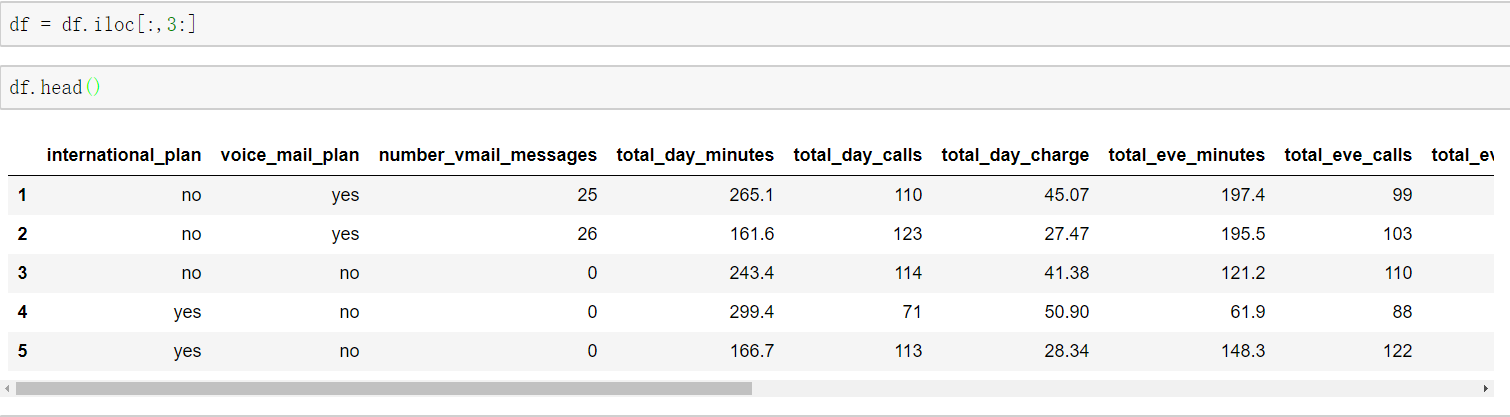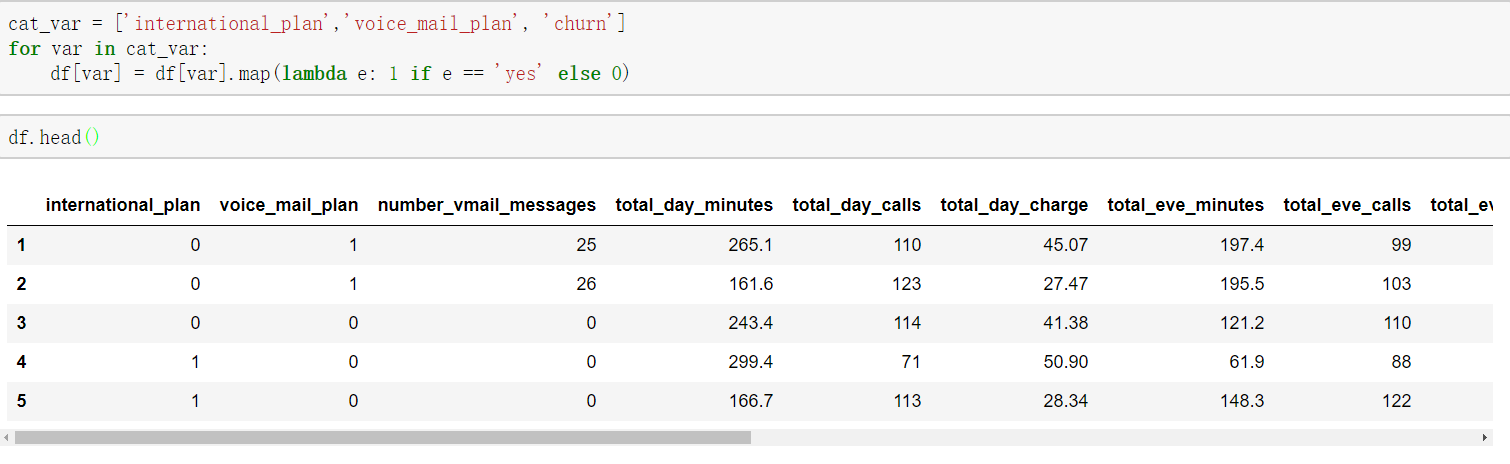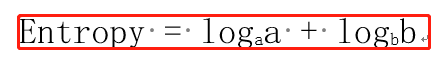### 三、使用机器学习建立分类模型

• 3.1使用决策树建立分类模型
• 3.1.1 模型建立

``````from sklearn import tree
# 将预测值（因变量）和影响参数列（自变量）分离开来。
y = df.iloc[:,-1]
X = df.iloc[:,:-1]
# max_depth指树的深度，一般来说深度越大准确度越高
clf1 = tree.DecisionTreeClassifier(max_depth=3)
# 将整理好的数据喂进去
clf1.fit(X, y)
``````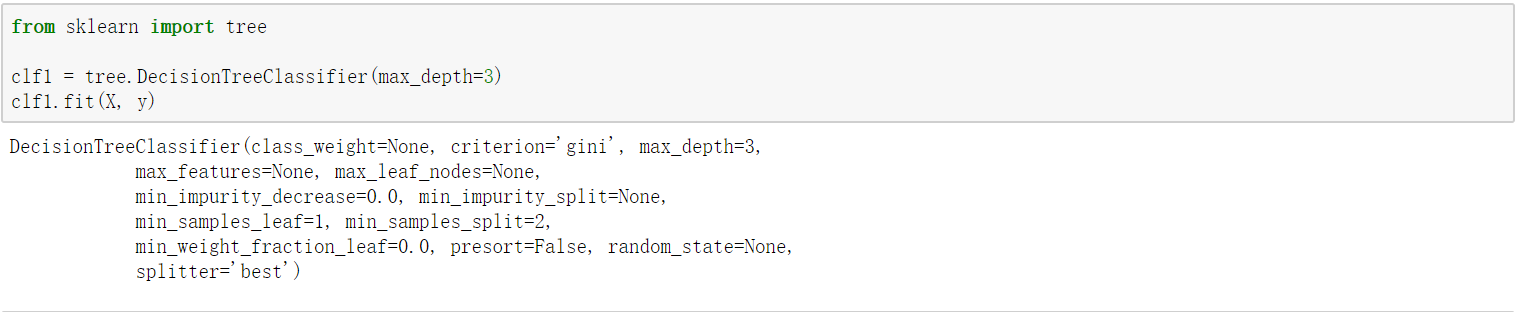• 3.1.2 模型可视化

``````# 将决策树输出为.dot文件，进而通过在命令行上使用dot tree.dot -Tpng -o tree.png 输出为.png图片，使用dot命令需安装graphviz软件，linux下直接apt-get install graphviz即可
tree.export_graphviz(clf0, out_file='tree.dot')
# 启用jupyter行内画
%pylab inline
from IPython.display import Image
Image('tree.png')``````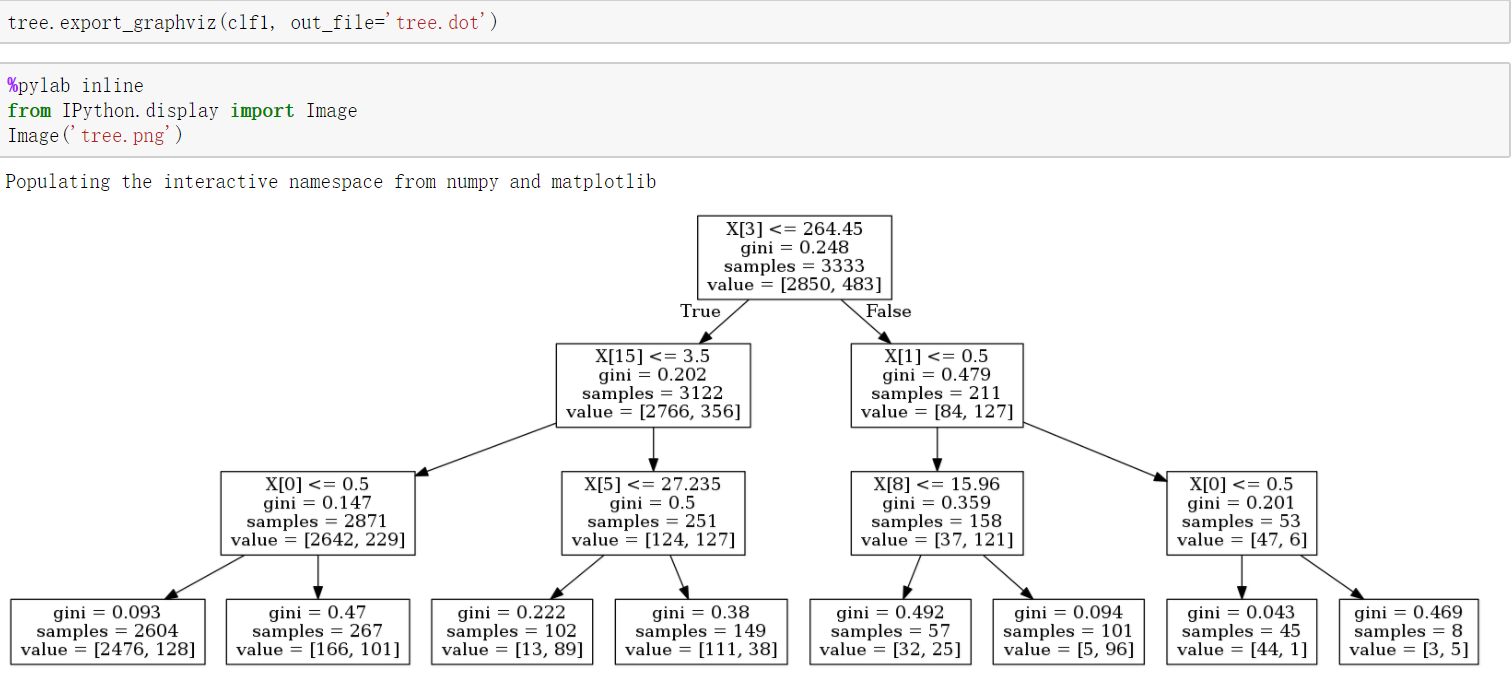• 3.1.3 模型结果

``````import numpy
# '假性'成功预测的概率率
numpy.sum(y== clf1.predict(X)) / len(y)``````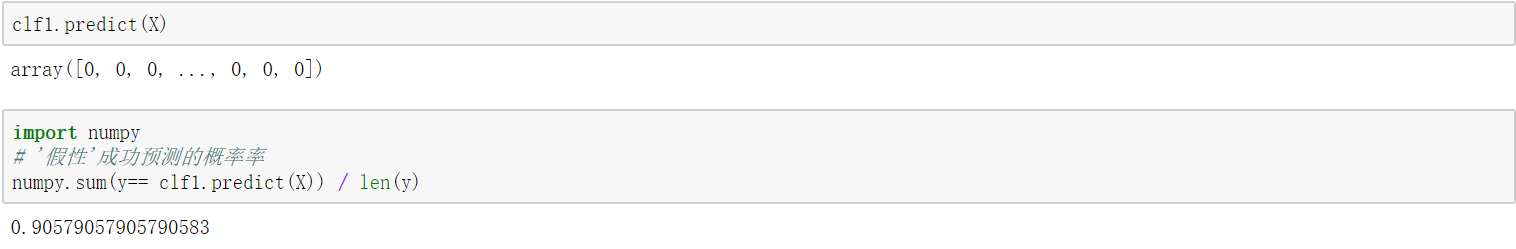• 3.2使用逻辑式回归建立分类模型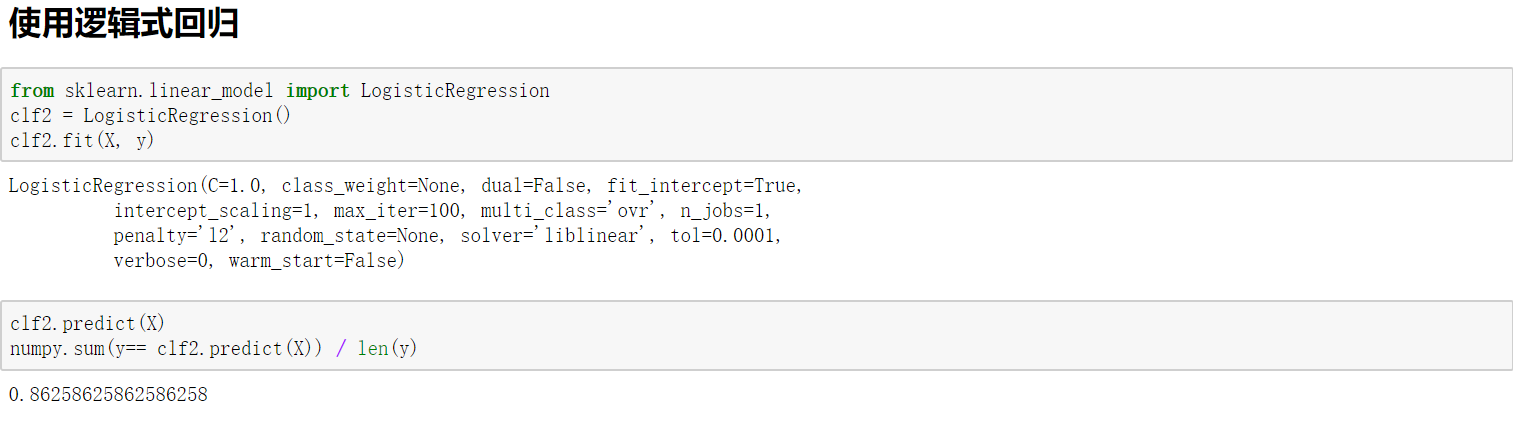• 3.3使用SVM建立分类模型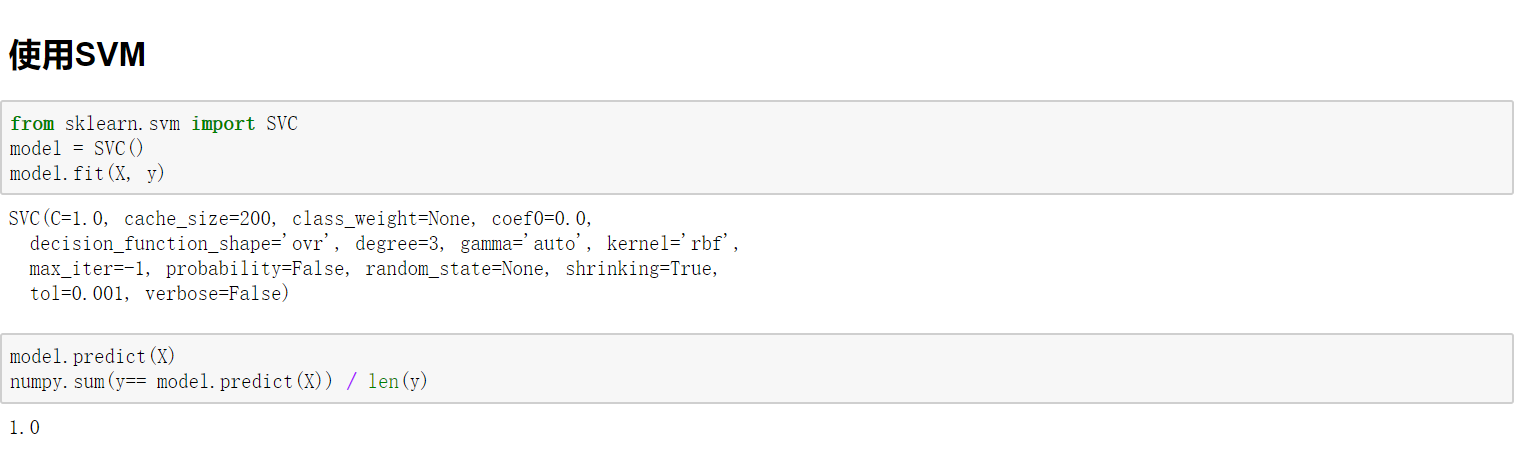• 3.4使用随机森林建立分类模型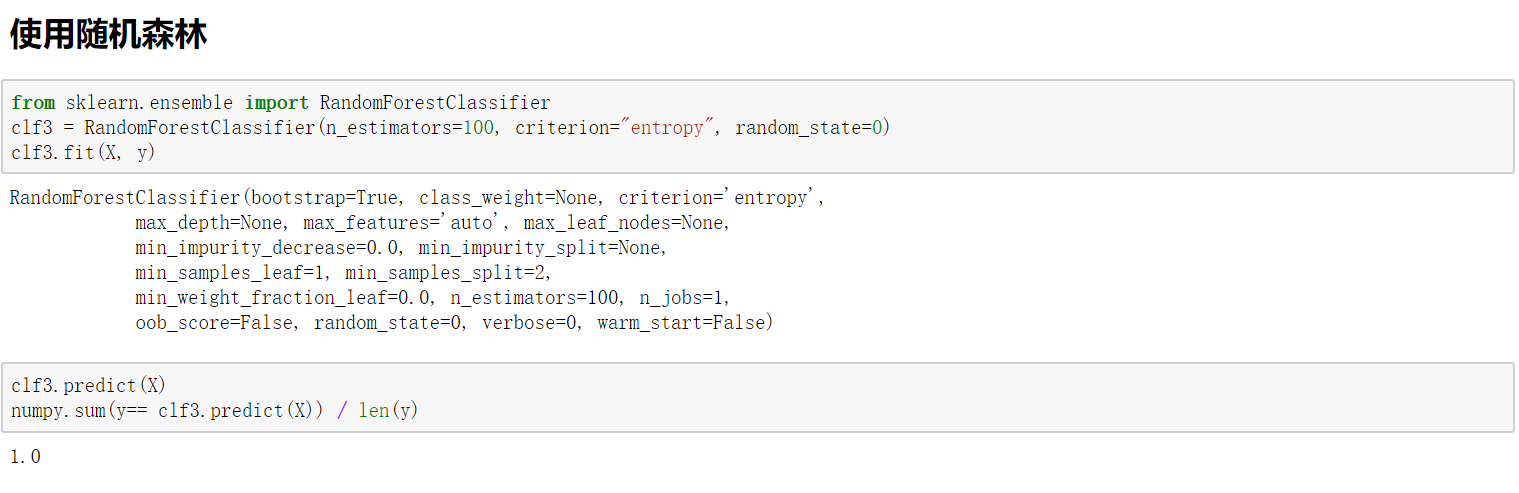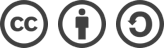0:00